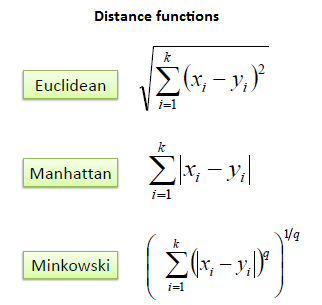/  Top Machine learning interview questions and answers   /  What are the Advantages and Disadvantages of KNN Classifier?## What are the Advantages and Disadvantages of KNN Classifier?

### Advantages of KNN

1. No Training Period: KNN is called Lazy Learner (Instance based learning). It does not learn anything in the training period. It does not derive any discriminative function from the training data. In other words, there is no training period for it. It stores the training dataset and learns from it only at the time of making real time predictions. This makes the KNN algorithm much faster than other algorithms that require training e.g. SVM, Linear Regression etc.

2. Since the KNN algorithm requires no training before making predictions, new data can be added seamlessly which will not impact the accuracy of the algorithm.

3. KNN is very easy to implement. There are only two parameters required to implement KNN i.e. the value of K and the distance function (e.g. Euclidean or Manhattan etc.)

### Disadvantages of KNN

1. Does not work well with large dataset: In large datasets, the cost of calculating the distance between the new point and each existing point is huge which degrades the performance of the algorithm.

2. Does not work well with high dimensions: The KNN algorithm doesn’t work well with high dimensional data because with large number of dimensions, it becomes difficult for the algorithm to calculate the distance in each dimension.

3. Need feature scaling: We need to do feature scaling (standardization and normalization) before applying KNN algorithm to any dataset. If we don’t do so, KNN may generate wrong predictions.

4. KNN is sensitive to noise in the dataset. We need to manually impute missing values and remove outliers.